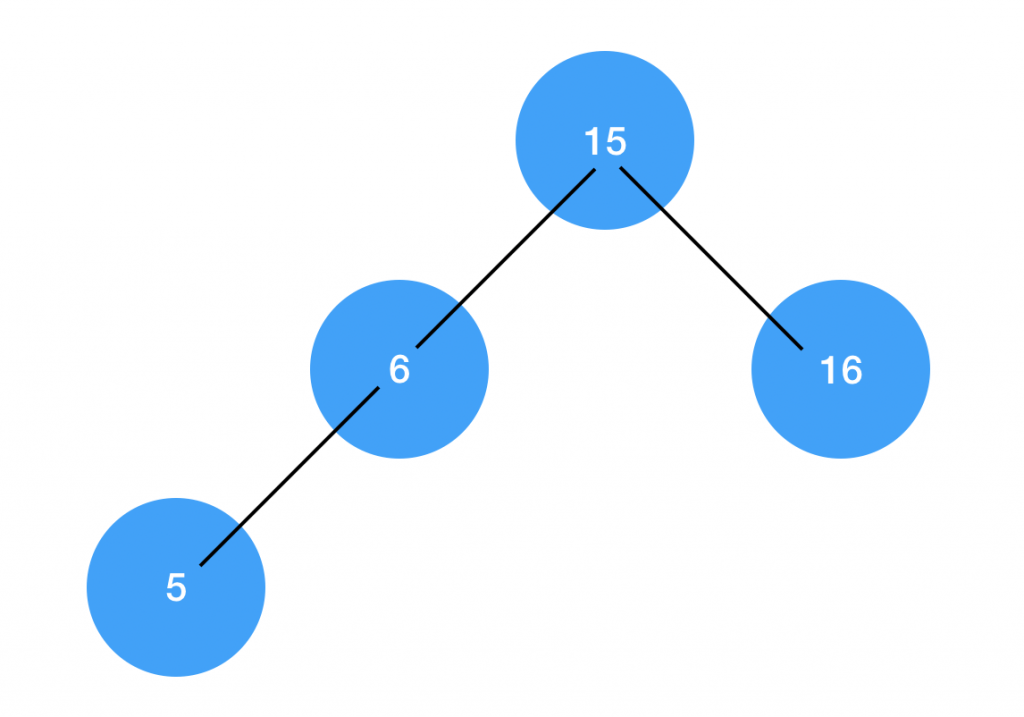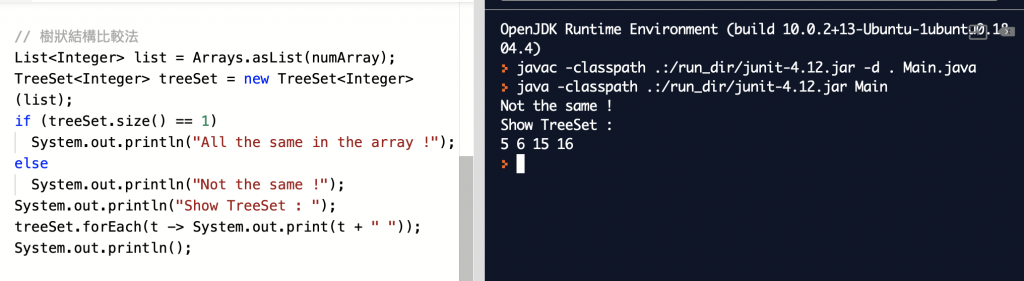#1

## 透過數學技巧改善程式效能系列-設計判斷式

`給定一個任意數字陣列 list，例如`
`15, 6, 15, 5, 15, 16`
`要求確認陣列中每一個數字是否都完全相同，要如何比較？`

``````// 固定將陣列的第一個值當作參考值
Integer check = 15;

int sumCheck = 0;
for (Integer i : list) {
if (i == check)

// 在迴圈中計數與參考值相同次數
++sumCheck;
}

// 如果計數次數等於陣列大小表示陣列裡面的值全同
if (sumCheck == list.size())
System.out.println("All the same in the array !");
else
System.out.println("Not the same !");
``````

`6 + 1` 次的 `if判斷` (迴圈裡6次 與 最後結論1次)。

``````兩點相同表示距離為 0 ，兩點重合
``````

`(15, 6, 15, 5, 15, 16) 與 (15, 15, 15, 15, 15, 15)`
`的距離是否為 0 ?`

``````int check = 15;
int sum = 0;
for (int i : numArray) {

// 兩點距離公式可省去開平方根動作
sum += (i-check)*(i-check);
}
if (sum == 0)
System.out.println("All the same in the array !");
else
System.out.println("Not the same !");
``````

``````sum += (i-check)*(i-check);
``````

`做 『加減乘除』 絕對比 『if判斷』 的工作輕鬆得多。```````// 陣列轉換成 TreeSet 步驟
Integer[] numArray = {15, 6, 15, 5, 15, 16};
List<Integer> list = Arrays.asList(numArray);
TreeSet<Integer> treeSet = new TreeSet<Integer>(list);

// 轉換成樹狀結構後，只要判斷樹狀結構是否為單一節點即為題目所求
if (treeSet.size() == 1)
System.out.println("All the same in the array !");
else
System.out.println("Not the same !");
```````數學的技巧就會派上用場了 ！`